TitleCollege Algebra
Answer/Discussion to Practice Problems
Tutorial 21: Absolute Value Equations

WTAMU > Virtual Math Lab > College Algebra > Tutorial 21: Absolute Value EquationsAnswer/Discussion to 1a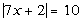We need to think about what value(s) are 10 units away from zero on the number line. Using the definition of absolute value there will be two equations that we will need to set up to get rid of the absolute value, because there are two places on the number line that are 10 units away from zero: 10 and -10.

 First equation: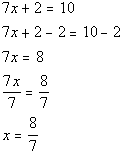*Setting inside linear expression = to 10 *Inv. of add. 2 is sub. 2   *Inv. of mult. by 7 is div. by 7

 Second equation: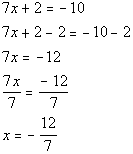*Setting inside linear expression = to -10 *Inv. of add. 2 is sub. 2   *Inv. of mult. by 7 is div. by 7

 When we plug 8/7 in for x, we end up with the absolute value of 10 which is 10.  When we plug -12/7 in for x, we end up with the absolute value of -10 which is also 10. If you would have only come up with an answer of x = 8/7, you would not have gotten all solutions to this problem. Again, it is important to note that we are using the definition of absolute value to set the two equations up.  Once you apply the definition and set it up without the absolute value, you just solve the linear equation as shown in Tutorial 14: Linear Equations in One Variable.  There are two solutions to this absolute value equation:  8/7 and -12/7.Answer/Discussion to 1b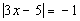Be careful on this one.  It is very tempting to set this up the same way we did problem 1 above, with two solutions.  However, note that the absolute value is set equal to a negative number.  There is no value of x that we can plug in that will be a solution because when we take the absolute value of the left side it will always be positive or zero, NEVER negative. Answer: No solution.Answer/Discussion to 1c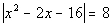We need to think about what value(s) are 8 units away from zero on the number line. Using the definition of absolute value, there will be two equations that we will need to set up to get rid of the absolute value because there are two places on the number line that are 8 units away from zero: 8 and -8.

 First equation: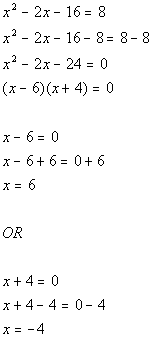*Setting inside quadratic expression = to 8 *Quad. eq. in standard form *Factor the trinomial *Use Zero-Product Principle *Set 1st factor = 0 and solve           *Set 2nd factor = 0 and solve

 Second equation: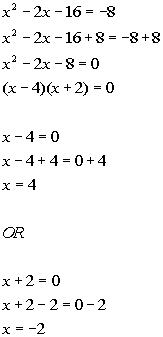*Setting inside quadratic expression = to -8 *Quad. eq. in standard form *Factor the trinomial *Use Zero-Product Principle *Set 1st factor = 0 and solve           *Set 2nd factor = 0 and solve

 When we plug 6 and -4 in for x, we end up with the absolute value of 8 which is 8.  When we plug 4 and -2 in for x, we end up with the absolute value of -8 which is also 8. If you would have only come up with an answer of x = 6 and -4, you would not have gotten all solutions to this problem. Again, it is important to note that we are using the definition of absolute value to set the two equations up.  Once you apply the definition and set it up without the absolute value, you just solve the quadratic equation as shown in Tutorial 17:  Quadratic Equations.  There are four solutions to this absolute value equation: 6, -4, 4, and -2.

WTAMU > Virtual Math Lab > College Algebra > Tutorial 21: Absolute Value Equations

Last revised on Dec. 16, 2009 by Kim Seward.
All contents copyright (C) 2002 - 2010, WTAMU and Kim Seward. All rights reserved.